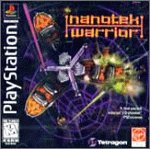# Nanotek Warrior cheats & more for PlayStation (PSX)

Get the latest Nanotek Warrior cheats, codes, unlockables, hints, Easter eggs, glitches, tips, tricks, hacks, downloads, hints, guides, FAQs, walkthroughs, and more for PlayStation (PSX). CheatCodes.com has all you need to win every game you play!

Use the above links or scroll down see all to the PlayStation cheats we have available for Nanotek Warrior.• Simulation, Space Combat Sim
• Unknown
• Virgin Interactive
• Unknown

## Hints

### Screen Shots

Insert the game disc into a PC compatible CD-ROM drive. Then, load the .JPG files with a graphics program to display screen shots from the game.

### Full Story

Insert the game disc into a PC compatible CD-ROM drive. Then go to the games folder and select the STORY.TXT file for the complete version of the NanoTek Warrior story.

## Cheats

### Destructible Obstacles

Pause the game and press Square, Circle, R2(2), Left, Up, Down, X. If you entered the code correctly, you will hear the power-up sound.

### Camera Lock

Pause the game and press Circle, Square, Triangle(2), Circle, Square, Triangle, Start. If you entered the code correctly, you will hear the power-up sound. Now the view will adjust to keep the ship in the center of the screen.

### Rotate Enemy And Obstacle Positions

Pause the game and press R1(2), Up, Circle, Square, Triangle, L2, X. If you entered the code correctly, you will hear the power-up sound. Now the next level played will have obstacles and enemies in a slightly different location from their original place.

### Cockpit View

Pause the game and press Triangle, Circle, Square(2), Triangle(2), Select, Start. If you entered the code correctly, you will hear the power-up sound. Now the view will switch to a semi-first person viewpoint.

### Random Curving

Pause the game and press Circle, Select, Left, Square(2), Down, Up, X. If you entered the code correctly, you will hear the power-up sound. Now the tube will curve randomly when the game is resumed.

### Stop Ship

Pause the game play press Triangle, Left, Triangle, Right, Triangle, Up, Triangle, Start. If you entered the code correctly, you will hear the power-up sound. Now press Triangle to stop your ship.

### Warp Speed

Pause the game and press Circle, Square, Circle, Square, Triangle(3), X. If you entered the code correctly, you will hear the power-up sound.

### Full Shield

Pause the game and press Select, Circle, Right, Up(2), L1(2), X. If you entered the code correctly, you will hear the power-up sound.

### Enable Memory Card

Insert a memory card before starting the game. Then, pause the game and press Left, Right, Left(2), R2, Circle, L1, X. If you entered the code correctly, you will hear the power-up sound. Now quit the game after completing level 2 or higher. An alternate password screen with five save game slots will appear.

### Black NanoTek Ship

Enter X, Square, X, Square, Square, Circle, X, Triangle, X as a password. Now the ship will be available with four lives and all weapons on level 1.

### Special Weapons

Enter X, Square, Triangle, Circle, Square, Circle, X, Triangle, X as a password. Entering X, Triangle, Circle, Square, Circle, X, Triangle, X as a password will also work.

### Level Passwords

Level Password Normal difficulty 2 Square, Triangle, X, Triangle, Square, X, Square, Triangle, X 3 Triangle, Square, X, Triangle, Square, Triangle, X, Square, X Bonus 1 Square, Circle, X, Triangle, Square, Circle, Triangle, Circle, X 4 X, Triangle, Square, X, Square, Triangle, X, Square, Circle 5 Circle, Triangle, Square, X, Square, Circle, X, Circle, Square 6 Triangle, Circle, Square, X, Square, Triangle, X, Circle, X Bonus 2 Square, Square, Square, X, Square, Square, Triangle, Triangle, Square 7 X, Triangle, X, Circle, Square, X, Triangle, Circle, Square 8 Square, Triangle, X, Circle, Square, X, Triangle, Circle, X Hard difficulty 2 Square, X, Triangle, X, Square, Triangle, X, Square, Circle 3 X, Square, Triangle, X, Square, Circle, X, Square, Triangle Bonus 1 Square, Circle, Triangle, X, Square, Triangle, Circle, Square, X 4 X, Triangle, Circle, X, Square, Triangle, Circle, Square, Triangle 5 Square, Triangle, Circle, X, Square, Triangle, X, Circle, X 6 Triangle, Square, Circle, Triangle, X, Circle, Square, Circle, Triangle Bonus 2 Circle, Square, Circle, Triangle, X, Triangle, Square, Circle, X 7 Triangle, X, Triangle, Circle, X, Square, X, Square, Triangle 8 Square, X, Triangle, Circle, X, Square, Triangle, Square, X

## Unlockables

We have no unlockables for Nanotek Warrior yet. If you have any unlockables please submit them.

## Easter eggs

We have no easter eggs for Nanotek Warrior yet. If you have any unlockables please submit them.

## Glitches

We have no glitches for Nanotek Warrior yet. If you have any unlockables please submit them.

## Guides

We have no guides or FAQs for Nanotek Warrior yet. If you have any unlockables please submit them.